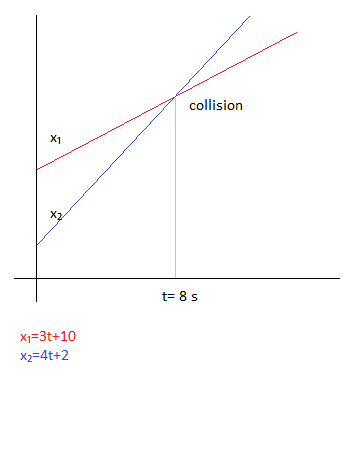# The equation for the location x (in meters) of a moving automobile at time t (in seconds) is x =...

## Question:

The equation for the location x (in meters) of a moving automobile at time t (in seconds) is x = 3t + 10.

What is the slope of the equation (include units) and what is its physical meaning?

What is the x-intercept in the above equation? What is its physical meaning?

What is the car's acceleration?

Would a second car with location x = 4 t + 2 ever collide with the first car? At what time? Give a graphical solution by making a sketch showing x versus t for both cars, and look for any intersect.

## Kinematics:

The velocity in position time graph is given by the slope of graph. The slope can by found by the derivative of the equation with respect to time. The acceleration of the car is the second derivative of position. The point of collision is the point of intersection of the two graphs.

Given:

{eq}x=3t+10 \\ \Rightarrow v= \dfrac{dx}{dt}=3\ m/s \\ {/eq}

The slope of the equation gives velocity.

For X intercept

put t=0

{eq}x=10 \\ {/eq} which is the position of automobile at t=0.

Acceleration of car is given by

{eq}a= \dfrac{d^2x}{dt^2}=0\ m/s^2 {/eq}

At the time of collision, both the cars are at the same position

{eq}3t+10=4t+2 \\ \Rightarrow t=8\ s {/eq}